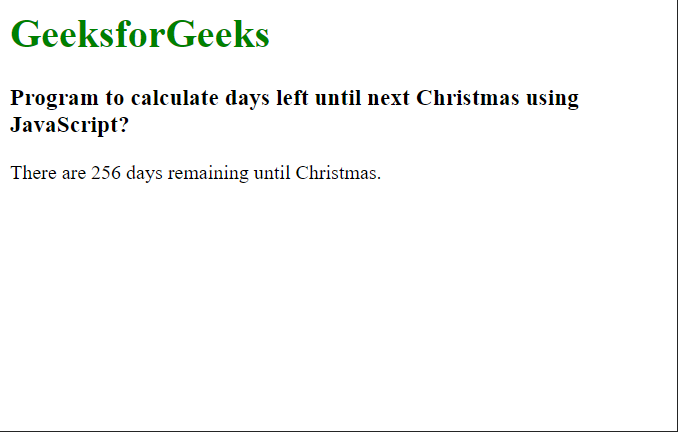Open in App
Not now

# How to calculate days left until next Christmas using JavaScript ?

• Difficulty Level : Hard
• Last Updated : 14 Apr, 2021

In this article, we will learn how to calculate the number of days left until next Christmas using JavaScript. Christmas marks the birth of Christ, mainly celebrated by millions of people around the globe on 25 December every year as a religious and cultural festival.

Approach: In order to calculate the number of days between two dates in JavaScript, the Date object must be used. We find out the year value of this year’s Christmas Day using getFullYear() method. We then check if the current date has already passed the Christmas day by checking if the month is December and the day is more than the 25th. The getMonth() method is used to get the month and the getDate() method is used to get the date value of the given time.

If this condition is satisfied, we add one more year to the Christmas year that we found earlier, hence advancing the Christmas Day for the next year. We then create the final date value of the next Christmas Day.

We can use the getTime() function to obtain both the date values in milliseconds. After the conversion, we subtract the later one from the earlier one to get the difference in milliseconds. The final number of days is obtained by dividing the difference (in milliseconds) between the two dates by the number of milliseconds in one day.

Syntax:

```let today = new Date();
let christmasYear = today.getFullYear();

if (today.getMonth() == 11 && today.getDate() > 25) {
christmasYear = christmasYear + 1;
}

let christmasDate = new Date(christmasYear, 11, 25);
let dayMilliseconds = 1000 * 60 * 60 * 24;

let remainingDays = Math.ceil(
(christmasDate.getTime() - today.getTime()) /
(dayMilliseconds)
);```

Example:

## HTML

 `<``html``> ` `<``body``> ` `  ``<``h1` `style``=``"color: green;"``> ` `    ``GeeksforGeeks ` `  `` ` `  ``<``h3``> ` `    ``Program to calculate days left until ` `    ``next Christmas using JavaScript? ` `  `` ` `  ``<``script``> ` `    ``// Get the current date ` `    ``let today = new Date(); ` ` `  `    ``// Get the year of the current date ` `    ``let christmasYear = today.getFullYear(); ` ` `  `    ``// Check if the current date is ` `    ``// already past by checking if the month ` `    ``// is December and the current day ` `    ``// is greater than 25 ` `    ``if (today.getMonth() == 11 && ` `        ``today.getDate() > 25) { ` ` `  `      ``// Add an year so that the next ` `      ``// Christmas date could be used ` `      ``christmasYear = christmasYear + 1; ` `    ``} ` ` `  `    ``// Get the date of the next Christmas ` `    ``let christmasDate =  ` `        ``new Date(christmasYear, 11, 25); ` ` `  `    ``// Get the number of milliseconds in 1 day ` `    ``let dayMilliseconds = ` `        ``1000 * 60 * 60 * 24; ` ` `  `    ``// Get the remaining amount of days ` `    ``let remainingDays = Math.ceil( ` `      ``(christmasDate.getTime() - today.getTime()) / ` `      ``(dayMilliseconds) ` `    ``); ` ` `  `    ``// Write it to the page ` `    ``document.write("There are " + remainingDays + ` `                   ``" days remaining until Christmas."); ` `  `` ` ` ` ``

Output:My Personal Notes arrow_drop_up
Related Articles